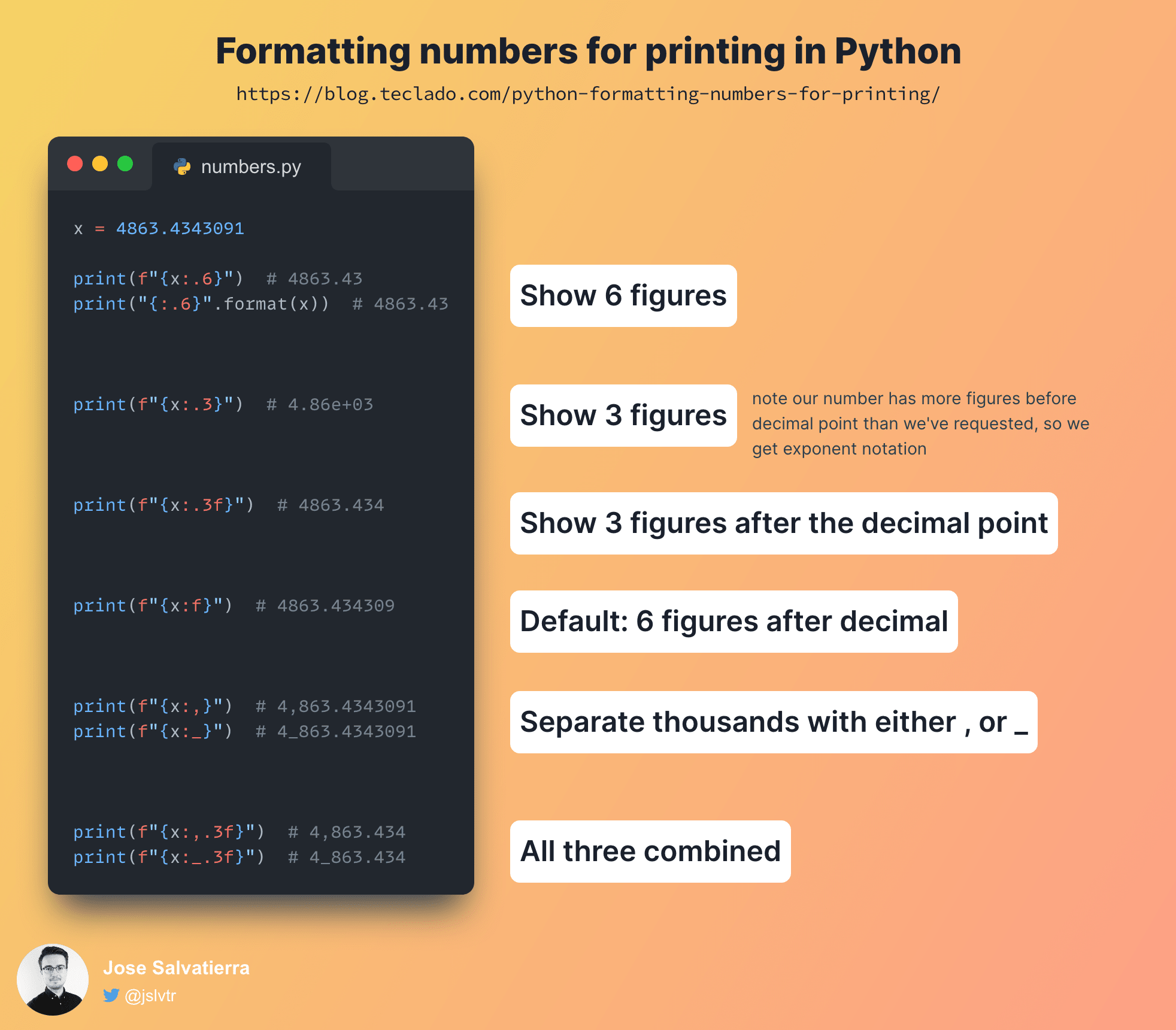Hey there, and welcome to another Python snippet post. Let's take a look at how to print formatted numbers. We'll cover rounding, thousands separators, and percentages.

Before we dive in, here's a quick image overview:String formatting is actually a surprisingly large topic, and Python has its own internal mini language just for handling the many formatting options available to us. Here we're just going to focus on a few examples involving numbers, but there is a great deal more to explore.

## Format numbers rounded to certain decimal places

First let's take a look at formatting a floating point number to a given level of precision. There are two ways we can do this: we can specify how many significant figures we want overall, or we can specify how many significant figures we want after the decimal point. Let's start with the former.

To specify a level of precision, we need to use a colon (`:`), followed by a decimal point, along with some integer representing the degree of precision. We place this inside the curly braces for an f-string, after the value we want to format. You can also use the `format` method instead, which I'll demonstrate below.

``````x = 4863.4343091          # example float to format

print(f"{x:.6}")          # f-string version
print("{:.6}".format(x))  # format method version
``````

In both cases we get the same result: `4863.43`.

As we can see, our very long float got shortened down to just 6 figures. An interesting thing to note is that this formatting operation actually performs rounding, so if `x` had a value of `4863.435`, the number printed would actually be `4863.44`. This uses bankers' rounding (explained here).

If we specify fewer figures than we have in the integer portion of the float, we end up with an exponent representation instead:

``````x = 4863.4343091

print(f"{x:.3}")  # 4.86e+03
``````

`4.86e+03` means `4.86 x 10³`, or `4.86 x 1000`, which is `4860`. Looking at this result, we see that we got three significant figures, as we requested.

So how do we specify 3 decimal places? We just need to add an `f`.

``````x = 4863.4343091

print(f"{x:.3f}")  # 4863.434
``````

`f` indicates that we want our float displayed as a "fixed point number": in other words, we want a specific number of digits after the decimal point. We can use `f` on its own as well, which defaults to 6 digits of precision:

``````x = 4863.4343091

print(f"{x:f}")  # 4863.434309
``````

## Display separator character between thousands

For large numbers we often write a separator character (usually a comma, space, or period) to make it easier to read. We can specify this in Python using a comma or underscore character after the colon.

``````x = 1000000

print(f"{x:,}")  # 1,000,000
print(f"{x:_}")  # 1_000_000
``````

This also works with floats, and the precision formatting, with the comma coming first:

``````x = 4863.4343091

print(f"{x:,.3f}")  # 4,863.434
print(f"{x:_.3f}")  # 4_863.434
``````

## Format floating point numbers as percentages

We can format a number as a percentage by simply adding the percent symbol at the end of our formatting options instead of `f`:

``````questions = 30